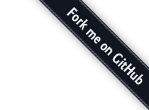• “表达式”（expression）是一个单纯的运算过程，总是有返回值；
• “语句”（statement）是执行某种操作，没有返回值。

``````function foo(){}; // 声明，因为它是程序的一部分
var bar = function foo(){}; // 表达式，因为它是赋值表达式的一部分

new function bar(){}; // 表达式，因为它是New表达式的一部分

(function(){
function bar(){}; // 声明，因为它是函数体的一部分
})();
``````

``````(function foo(){}); // 函数表达式：注意它被包含在分组操作符中
(var x = 5);  // error! 分组操作符只能包含表达式，不能包含语句（这里的var就是语句）
``````

``````alert(eval(data));
``````

``````{"username" : "justjavac"}
``````

``````{
}
``````

``````({"username" : "justjavac"})
``````

``````eval('(' + json + ')')
``````

``````(var a = 4) + 4;
``````

``````if (var a = 0) {}
``````

``````if (expression) {
statement;
statement;
……
}
``````

``````if (var a = 0) {}
``````

``````if (true) {
var a = 0;
}
``````